# 4.6 Special binomial products

 Page 1 / 1
This module is from Elementary Algebra by Denny Burzynski and Wade Ellis, Jr. Operations with algebraic expressions and numerical evaluations are introduced in this chapter. Coefficients are described rather than merely defined. Special binomial products have both literal and symbolic explanations and since they occur so frequently in mathematics, we have been careful to help the student remember them. In each example problem, the student is "talked" through the symbolic form.Objectives of this module: be able to expand (a + b)^2, (a - b)^2, and (a + b)(a - b).

## Overview

• Expanding ${\left(a+b\right)}^{2}$ and ${\left(a-b\right)}^{2}$
• Expanding $\left(a+b\right)\left(a-b\right)$

Three binomial products occur so frequently in algebra that we designate them as special binomial products . We have seen them before (Sections [link] and [link] ), but we will study them again because of their importance as time saving devices and in solving equations (which we will study in a later chapter).

These special products can be shown as the squares of a binomial

${\left(a+b\right)}^{2}$      and      ${\left(a-b\right)}^{2}$

and as the sum and difference of two terms .

$\left(a+b\right)\left(a-b\right)$

There are two simple rules that allow us to easily expand (multiply out) these binomials. They are well worth memorizing, as they will save a lot of time in the future.

## Squaring a binomial

To square a binomial: $*$

1. Square the first term.
2. Take the product of the two terms and double it.
3. Square the last term.
4. Add the three results together.

$\begin{array}{c}{\left(a+b\right)}^{2}={a}^{2}+2ab+{b}^{2}\\ {\left(a-b\right)}^{2}={a}^{2}-2ab+{b}^{2}\end{array}$

## Sum and difference of two terms

To expand the sum and difference of two terms: $†$

1. Square the first term and square the second term.
2. Subtract the square of the second term from the square of the first term.

$\left(a+b\right)\left(a-b\right)={a}^{2}-{b}^{2}$

$*$ See problems 56 and 57 at the end of this section.
$†$ See problem 58.

## Sample set a

Note that ${\left(x+4\right)}^{2}\ne {x}^{2}+{4}^{2}$ . The $8x$ term is missing!

Notice that the sign of the last term in this expression is “ $+$ .” This will always happen since the last term results from a number being squared . Any nonzero number times itself is always positive.

The sign of the second term in the trinomial will always be the sign that occurs inside the parentheses.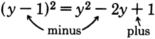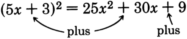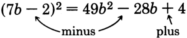$\begin{array}{ll}\left(x+6\right)\left(x-6\right)\hfill & \text{Square}\text{\hspace{0.17em}}\text{the}\text{\hspace{0.17em}}\text{first}\text{\hspace{0.17em}}\text{term:}\text{\hspace{0.17em}}\text{\hspace{0.17em}}\text{\hspace{0.17em}}\text{\hspace{0.17em}}\text{\hspace{0.17em}}{x}^{2}.\hfill \\ \hfill & \text{Subtract}\text{\hspace{0.17em}}\text{the}\text{\hspace{0.17em}}\text{square}\text{\hspace{0.17em}}\text{of}\text{\hspace{0.17em}}\text{the}\text{\hspace{0.17em}}\text{second}\text{\hspace{0.17em}}\text{term}\text{\hspace{0.17em}}\left(36\right)\text{\hspace{0.17em}}\text{from}\hfill \\ \hfill & \text{the}\text{\hspace{0.17em}}\text{square}\text{\hspace{0.17em}}\text{of}\text{\hspace{0.17em}}\text{the}\text{\hspace{0.17em}}\text{first}\text{\hspace{0.17em}}\text{term:}\text{\hspace{0.17em}}\text{\hspace{0.17em}}\text{\hspace{0.17em}}\text{\hspace{0.17em}}{x}^{2}-36.\hfill \\ \left(x+6\right)\left(x-6\right)={x}^{2}-36\hfill & \hfill \end{array}$

$\begin{array}{ll}\left(4a-12\right)\left(4a+12\right)\hfill & \text{Square}\text{\hspace{0.17em}}\text{the}\text{\hspace{0.17em}}\text{first}\text{\hspace{0.17em}}\text{term:}\text{\hspace{0.17em}}\text{\hspace{0.17em}}\text{\hspace{0.17em}}\text{\hspace{0.17em}}\text{\hspace{0.17em}}16{a}^{2}.\hfill \\ \hfill & \text{Subtract}\text{\hspace{0.17em}}\text{the}\text{\hspace{0.17em}}\text{square}\text{\hspace{0.17em}}\text{of}\text{\hspace{0.17em}}\text{the}\text{\hspace{0.17em}}\text{second}\text{\hspace{0.17em}}\text{term}\text{\hspace{0.17em}}\left(144\right)\text{\hspace{0.17em}}\text{from}\hfill \\ \hfill & \text{the}\text{\hspace{0.17em}}\text{square}\text{\hspace{0.17em}}\text{of}\text{\hspace{0.17em}}\text{the}\text{\hspace{0.17em}}\text{first}\text{\hspace{0.17em}}\text{term:}\text{\hspace{0.17em}}\text{\hspace{0.17em}}\text{\hspace{0.17em}}\text{\hspace{0.17em}}16{a}^{2}-144.\hfill \\ \left(4a-12\right)\left(4a+12\right)=16{a}^{2}-144\hfill & \hfill \end{array}$

$\begin{array}{ll}\left(6x+8y\right)\left(6x-8y\right)\hfill & \text{Square}\text{\hspace{0.17em}}\text{the}\text{\hspace{0.17em}}\text{first}\text{\hspace{0.17em}}\text{term:}\text{\hspace{0.17em}}\text{\hspace{0.17em}}\text{\hspace{0.17em}}\text{\hspace{0.17em}}\text{\hspace{0.17em}}36{x}^{2}.\hfill \\ \hfill & \text{Subtract}\text{\hspace{0.17em}}\text{the}\text{\hspace{0.17em}}\text{square}\text{\hspace{0.17em}}\text{of}\text{\hspace{0.17em}}\text{the}\text{\hspace{0.17em}}\text{second}\text{\hspace{0.17em}}\text{term}\text{\hspace{0.17em}}\left(64{y}^{2}\right)\text{\hspace{0.17em}}\text{from}\hfill \\ \hfill & \text{the}\text{\hspace{0.17em}}\text{square}\text{\hspace{0.17em}}\text{of}\text{\hspace{0.17em}}\text{the}\text{\hspace{0.17em}}\text{first}\text{\hspace{0.17em}}\text{term:}\text{\hspace{0.17em}}\text{\hspace{0.17em}}\text{\hspace{0.17em}}\text{\hspace{0.17em}}36{x}^{2}-64{y}^{2}.\hfill \\ \left(6x+8y\right)\left(6x-8y\right)=36{x}^{2}-64{y}^{2}\hfill & \hfill \end{array}$

## Practice set a

Find the following products.

${\left(x+5\right)}^{2}$

${x}^{2}+10x+25$

${\left(x+7\right)}^{2}$

${x}^{2}+14x+49$

${\left(y-6\right)}^{2}$

${y}^{2}-12y+36$

${\left(3a+b\right)}^{2}$

$9{a}^{2}+6ab+{b}^{2}$

${\left(9m-n\right)}^{2}$

$81{m}^{2}-18mn+{n}^{2}$

${\left(10x-2y\right)}^{2}$

$100{x}^{2}-40xy+4{y}^{2}$

${\left(12a-7b\right)}^{2}$

$144{a}^{2}-168ab+49{b}^{2}$

${\left(5h-15k\right)}^{2}$

$25{h}^{2}-150hk+225{k}^{2}$

## Exercises

For the following problems, find the products.

${\left(x+3\right)}^{2}$

${x}^{2}+6x+9$

${\left(x+5\right)}^{2}$

${\left(x+8\right)}^{2}$

${x}^{2}+16x+64$

${\left(x+6\right)}^{2}$

${\left(y+9\right)}^{2}$

${y}^{2}+18y+81$

${\left(y+1\right)}^{2}$

${\left(a-4\right)}^{2}$

${a}^{2}-8a+16$

${\left(a-6\right)}^{2}$

${\left(a-7\right)}^{2}$

${a}^{2}-14a+49$

${\left(b+10\right)}^{2}$

${\left(b+15\right)}^{2}$

${b}^{2}+30b+225$

${\left(a-10\right)}^{2}$

${\left(x-12\right)}^{2}$

${x}^{2}-24x+144$

${\left(x+20\right)}^{2}$

${\left(y-20\right)}^{2}$

${y}^{2}-40y+400$

${\left(3x+5\right)}^{2}$

${\left(4x+2\right)}^{2}$

$16{x}^{2}+16x+4$

${\left(6x-2\right)}^{2}$

${\left(7x-2\right)}^{2}$

$49{x}^{2}-28x+4$

${\left(5a-6\right)}^{2}$

${\left(3a-9\right)}^{2}$

$9{a}^{2}-54a+81$

${\left(3w-2z\right)}^{2}$

${\left(5a-3b\right)}^{2}$

$25{a}^{2}-30ab+9{b}^{2}$

${\left(6t-7s\right)}^{2}$

${\left(2h-8k\right)}^{2}$

$4{h}^{2}-32hk+64{k}^{2}$

${\left(a+\frac{1}{2}\right)}^{2}$

${\left(a+\frac{1}{3}\right)}^{2}$

${a}^{2}+\frac{2}{3}a+\frac{1}{9}$

${\left(x+\frac{3}{4}\right)}^{2}$

${\left(x+\frac{2}{5}\right)}^{2}$

${x}^{2}+\frac{4}{5}x+\frac{4}{25}$

${\left(x-\frac{2}{3}\right)}^{2}$

${\left(y-\frac{5}{6}\right)}^{2}$

${y}^{2}-\frac{5}{3}y+\frac{25}{36}$

${\left(y+\frac{2}{3}\right)}^{2}$

${\left(x+1.3\right)}^{2}$

${x}^{2}+2.6x+1.69$

${\left(x+5.2\right)}^{2}$

${\left(a+0.5\right)}^{2}$

${a}^{2}+a+0.25$

${\left(a+0.08\right)}^{2}$

${\left(x-3.1\right)}^{2}$

${x}^{2}-6.2x+9.61$

${\left(y-7.2\right)}^{2}$

${\left(b-0.04\right)}^{2}$

${b}^{2}-0.08b+0.0016$

${\left(f-1.006\right)}^{2}$

$\left(x+5\right)\left(x-5\right)$

${x}^{2}-25$

$\left(x+6\right)\left(x-6\right)$

$\left(x+1\right)\left(x-1\right)$

${x}^{2}-1$

$\left(t-1\right)\left(t+1\right)$

$\left(f+9\right)\left(f-9\right)$

${f}^{2}-81$

$\left(y-7\right)\left(y+7\right)$

$\left(2y+3\right)\left(2y-3\right)$

$4{y}^{2}-9$

$\left(5x+6\right)\left(5x-6\right)$

$\left(2a-7b\right)\left(2a+7b\right)$

$4{a}^{2}-49{b}^{2}$

$\left(7x+3t\right)\left(7x-3t\right)$

$\left(5h-2k\right)\left(5h+2k\right)$

$25{h}^{2}-4{k}^{2}$

$\left(x+\frac{1}{3}\right)\left(x-\frac{1}{3}\right)$

$\left(a+\frac{2}{9}\right)\left(a-\frac{2}{9}\right)$

${a}^{2}-\frac{4}{81}$

$\left(x+\frac{7}{3}\right)\left(x-\frac{7}{3}\right)$

$\left(2b+\frac{6}{7}\right)\left(2b-\frac{6}{7}\right)$

$4{b}^{2}-\frac{36}{49}$

Expand ${\left(a+b\right)}^{2}$ to prove it is equal to ${a}^{2}+2ab+{b}^{2}$ .

Expand ${\left(a-b\right)}^{2}$ to prove it is equal to ${a}^{2}-2ab+{b}^{2}$ .

$\left(a-b\right)\left(a-b\right)={a}^{2}-ab-ab+{b}^{2}={a}^{2}-2ab+{b}^{2}$

Expand $\left(a+b\right)\left(a-b\right)$ to prove it is equal to ${a}^{2}-{b}^{2}$ .

Fill in the missing label in the equation below.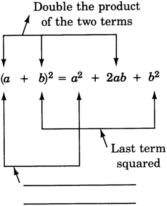first term squared

Label the parts of the equation below.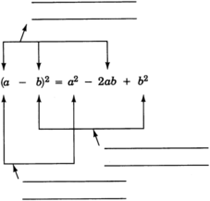Label the parts of the equation below.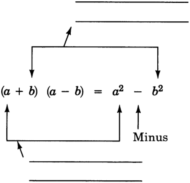(a) Square the first term.
(b) Square the second term and subtract it from the first term.

## Exercises for review

( [link] ) Simplify ${\left({x}^{3}{y}^{0}{z}^{4}\right)}^{5}$ .

( [link] ) Find the value of ${10}^{-1}\cdot {2}^{-3}$ .

$\frac{1}{80}$

( [link] ) Find the product. $\left(x+6\right)\left(x-7\right)$ .

( [link] ) Find the product. $\left(5m-3\right)\left(2m+3\right)$ .

$10{m}^{2}+9m-9$

( [link] ) Find the product. $\left(a+4\right)\left({a}^{2}-2a+3\right)$ .

What is scarcity.
Fiona
why our wants are limited
nooo want is unlimited but resources are limited
Ruchi
and do to that there occurs scarcity and we have to make choice in order to have what we need if need be I will explain more
our wants are not limited but rather the resources
Moses
as we know that there are two principle of microeconomics scarcity of resources and they have alternative uses...
Ruchi
yes .....
Mathias
because our resources are limited./we have a limited resources.
Ijeoma
what is demand
demand is something wt we called in economic theory of demand it simply means if price of product is increase then demand of product will decrease
Ruchi
inverse relationship between demand and price
Ruchi
in microeconomic
Ruchi
demand is what and how much you want and what's your need...
Shikhar
how can one be so with economics even while you have less knowledge in mathematics.
why is it that some products increases everyday by day
because demand is increase
Ruchi
because demand is increase
Patience
but how demand increases?
Aziz
Because of the Marketing and purchasing power of people.
AmarbirSingh
but how could we know that people's demands have increased everyday by day and how could we know that this is time to produced the products in the market. Is any connection among them
yaqoob
for normal good people demand remain the same if price of product will increase or not
Ruchi
see that some product which increases day by day is comes under normal good which is used by consumer
Ruchi
Seems hot discussing going here
Shamamet
If there are less products demand starts to increase for those products
Shamamet
Economics is really interesting to learn ....
Shamamet
see there is Inferior goods ands normal goods inferior good demand is rarely increase whereas as we talk about normal good demand will absolutely Increase whether price is increase or not
Ruchi
and demand for normal goods increase cause people's income as a while increases time to time
Abhisek
and it might also be that the cost of raw materials are high.
ATTAH
may be
Ruchi
obviously because demand is increasing.....and price is getting low.....
Shikhar
hmmm there is inverse relationship between demand and price
Ruchi
This is because the supply of those products in relation to raw materials are decreasing and they are also necessities. This crate shortage in the market, so sellers will rise the prices of those products.
Abdul
Importance of economics
the nature and significance of economics studies
Deborah
What is demand
deman is amount of goods and services a consumer is willing and able to buy or purchase at a given price.
Sainabou
the willingness and ability of a body to purchase goods nd servicesbis called demand ,so if she/has ability but doesn't have willingness it's not a demand same if she or he has willingness but doesn't has ability it's not a demand too
Gul
Demand refers to as quantities of a goods and services in which consumers are willing and able to purchase at a given period of time and demand can also be defined as the desire or willingness and backed by the ability to pay.
Yeah
Mathias
What is Choice
Kofi
Choice refers to the ability of a consumer or producer to decide which good, service or resource to purchase or provide from a range of possible options. Being free to chose is regarded as a fundamental indicator of economic well being and development.
Shonal
choice is a act of selecting or choosing from the numerous or plenty wants.
demand is want and it is also what you need and able to afford a particular period of time... because demand changes with time.
Ijeoma
Demand refers to the ability of the consumer to pay for a particular product at a given price
Abdul
how does consumer make profit
Ijeoma
Compare and contract the function of commercial bank and the central bank of Nigeria
what do think is the difference between overhead costs and prime cost
Abdoulkarim
what is economics
economics is a social science that study's how resources can be used to produce goods and services for society
Nathan
Economic is a science which studies human behavior as a relationship between ends and scares means which have alternatives uses or purposes.
what is economics
what is the basic economic problem
rules
unlimited wants vs limited resources
Nathan
what is a new paradigm shift
Paradigm shift it is the reconcilliation of fedural goods in production
Shyline
fedural? what is that?
Aziz
factors that affecting economic system
crux
Shyline
what is meant by broadening the tax base?
Difference between extinct and extici spicies
Please keep in mind that it's not allowed to promote any social groups (whatsapp, facebook, etc...), exchange phone numbers, email addresses or ask for personal information on QuizOver's platform.

#### Get Jobilize Job Search Mobile App in your pocket Now!ByByBy OpenStaxBy Kimberly NicholsBy OpenStaxBy Jonathan LongBy Madison ChristianBy Rachel WoolardBy Gerr ZenBy Madison ChristianBy Brooke DelaneyBy JavaChamp Team